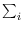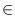Next: Dynamic Random Variates ( Up: Simple Data Types and Previous: Random Sources ( random_source   Contents   Index

# Random Variates ( random_variate )

Definition

An instance R of the data type random_variate is a non-uniform random number generator. The generation process is governed by an array<int> w. Let [l..r] be the index range of w and let W =w[i] be the total weight. Then any integer i[l..h] is generated with probability w[i]/W. The weight function w must be non-negative and W must be non-zero.

#include < LEDA/core/random_variate.h >

Creation

 random_variate R(const array& w) creates an instance R of type random_variate.

Operations

 int R.generate() generates i[l..h] with probability w[i]/W.